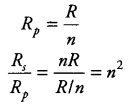# N resistors, each of resistance R are first connected in series and then in parallel

n resistors, each of resistance R are first connected in series and then in parallel. What is the ratio of the total effective resistance of the circuit in series to parallel combination?

In series combination, R_{s} = nR. In parallel combination,